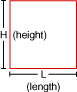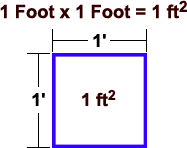Directions: Enter any combination of feet and/or inches into the calculator below. You can use this square footage calculator to calculate siding square footage or any other square feet needed (like: roofing, carpet, etc.) You can also use this tool to convert feet to inches, convert inches to feet and calculate square inches.rectangle examples
and diagram

# Square Footage Calculator

(Height x Length = Square Footage)
(Square Footage = Square Feet = SqFt = Sq.Ft. = sq.ft. = ft2)
(

## Height

feet     inches)
X
(

feet     inches)

## Directions:

Use the calculator above to calculate your square footage.
You can enter feet only, inches only or any combination of the two.
*Square Footage is also known as (a.k.a) square feet, square ft, SqFt, Sq.Ft., sq.ft., ft2 & ft2
**This Calculator also calculates square inches, total height in feet, total height in inches, total length in feet and total length in inches.## What is a Square Foot?

A "square foot" is a unit of area measurement equal to a square measuring one foot on each side. 1 Square Foot = 0.0929 square meters. Abbrev.: ft2, sq.ft, SqFt.